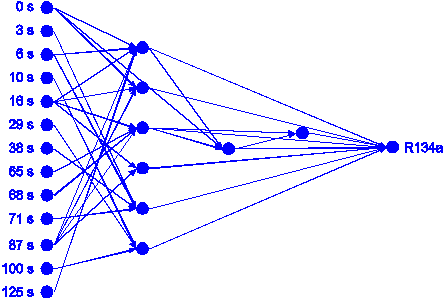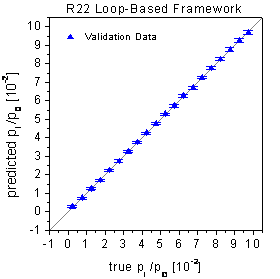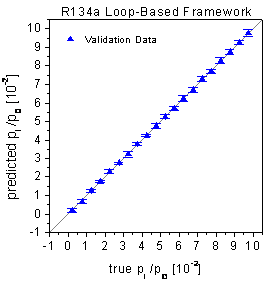Home News About Me Ph. D. Thesis Abstract Table of Contents 1. Introduction 2. Theory  Fundamentals of the Multivariate Data Analysis 3. Theory  Quantification of the Refrigerants R22 and R134a: Part I 4. Experiments, Setups and Data Sets 5. Results  Kinetic Measurements 6. Results  Multivariate Calibrations 7. Results  Genetic Algorithm Framework 8. Results  Growing Neural Network Framework 8.1. Modifications of the Growing Neural Network Algorithm 8.2. Application of the Growing Neural Networks 8.3. Growing Neural Network Algorithm Frameworks 8.4. Applications of the Growing Neural Network Frameworks 8.4.1. Parallel Framework 8.4.2. Loop-based Framework 8.5. Conclusions and Comparison of the Different Methods 9. Results  All Data Sets 10. Results  Various Aspects of the Frameworks and Measurements 11. Summary and Outlook 12. References 13. Acknowledgements Publications Research Tutorials Downloads and Links Contact Search Site Map Print this Page### 8.4.2.   Loop-based Framework

The loop-based approach was performed according to the flow chart shown in figure 54, whereby 10 neural networks were grown in each loop cycle. The complete calibration data set was split into training (60 %), monitor (20 %) and selection (20 %) subsets for each loop cycle. For R22 the framework stopped after 8 loop cycles with a network topology consisting of 16 input neurons, 56 links and 15 hidden neurons organized in 4 hidden layers shown in figure 57. For R134a the framework stopped after 7 loop cycles with a network topology consisting of 13 input neurons, 35 links and 8 hidden neurons organized in 3 hidden layers shown in figure 58. The predictions of the validation data by these network topologies show the best results of all multivariate calibration methods used for this data set with relative errors of 1.50% for R22 and 2.37% for R134a (see table 4). The true-predicted plots show no bias and very low standard deviations for all concentration levels (see figure 59). Compared with the parallel approach the loop-based network topologies use rather many input variables. It is also remarkable that the number of 3 respectively 4 layers of hidden neurons is unusually high. Yet, the non-uniform network design helps to keep the number of adjustable parameters low by building a sparse network topology with only few links. The topologies of the grown neural networks show that the common recommendation ,- to use only 1 or at the furthest 2 hidden layers for fully connected networks is only a vague rule, as the growing neural network algorithm automatically decides, how many hidden layers are optimal. The good generalization ability demonstrates that the non-uniform topology efficiently uses small networks and is superior to fully connected networks.

The same test for chance correlation and reproducibility was performed for the loop-based approach as already described for the genetic algorithm framework. Thereby the network topologies are by far more reproducible than using single runs of the growing neural network algorithm. The network for R22 of the second run uses the same variables than the network of the first run except of one variable being not used. The network for R134a uses the same variables of the first run except of one variable, which was exchanged by another one. Both networks did not use a random variable, whereby inside a loop cycle some networks used a random variable but these networks were not selected for the next loop cycle due to worse predictions of the selection data sets.figure 57:  Neural network with 4 hidden layers built by the loop-based framework for R22.figure 58:  Neural network with 3 hidden layers built by the loop-based framework for R134a.figure 59:  Predictions of the validation data by neural networks optimized by the loop-based growing network framework.

 Page 111 © Frank Dieterle, 03.03.2019